### Home > CALC > Chapter 2 > Lesson 2.2.3 > Problem2-85

2-85.
1. Jamal wrote the following Riemann sum to estimate the area under f(x) = 3x2 − 2. Homework Help ✎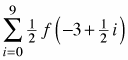1. Draw a sketch of the region. How many rectangles did he use?

2. For what domain of f(x) did Jamal estimate the area?

3. Use the summation feature of your calculator to find the approximate area using Jamal's Riemann sum.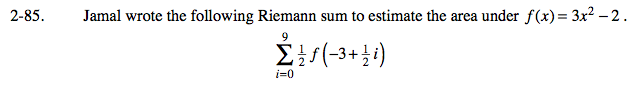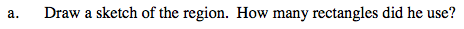General form of left-endpoint Riemann Sum:

$\sum_{i=0}^{n-1}\Delta xf(a+\Delta xi)$

n − 1 = 9 n = 10 There are 10 rectangles.

The function is f(x) = 3x² − 2

a = −3 This is where the region starts.

$\Delta x=\frac{1}{2}$

$\text{Each rectangle has a width of }\frac{1}{2}.$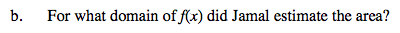Refer to the graph in part (a).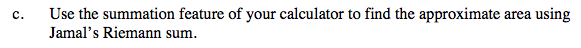Use the eTool below to complete the problem.
Click on the link to the right to view the full version of the eTool. Calc 2-85 HW eTool